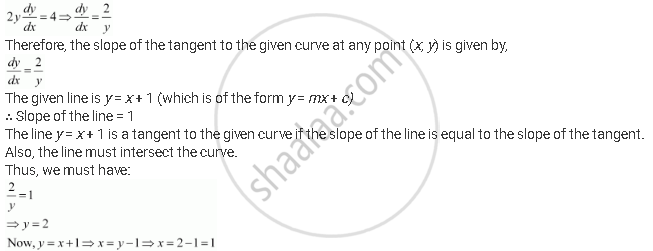Share

# The Line Y = X + 1 is a Tangent to the Curve Y2 = 4x At the Point - CBSE (Science) Class 12 - Mathematics

#### Question

The line y = x + 1 is a tangent to the curve y2 = 4x at the point

(A) (1, 2)

(B) (2, 1)

(C) (1, −2)

(D) (−1, 2)

#### Solution

The equation of the given curve is y2 = 4x

Differentiating with respect to x, we have:Hence, the line y = x + 1 is a tangent to the given curve at the point (1, 2).